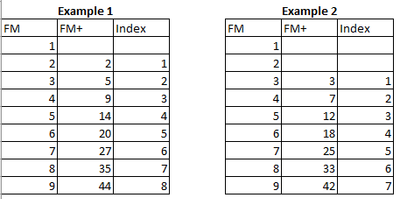cancel
Showing results for
Did you mean:Helper IV

## Dynamic Index

Hi,

I would like to add an index number with a measure.

When column FM+ (=Measure) has an value then start index with 1.

How can i achieve this?1 ACCEPTED SOLUTIONMemorable Member

The code below will start the Index column at the first Non-Null in the FM+ column.

Read the code comments as the code can be simplified if nulls will ONLY be at the beginning of the column and not interspersed within the column.

Since it does not depend on a Join (merge), there is no issue with duplicates.

``````let
Source = Table.FromColumns(
{{1..9},
{null,null, 2,5,9,14,null,27,35}},
type table[FM=Int64.Type, #"FM+"=Int64.Type]),

//Add index starting at first non-blank entry in FM+

//use next line if there will never be nulls except at the beginning of the column
//    #"FM+ 1st" = Table.RowCount(Source) - List.NonNullCount(Source[#"FM+"]),

//if nulls could be anywhere but we need to start at the First non-null then
#"FM+ 1st" =
List.Max(
List.Accumulate(
List.PositionOf(Source[#"FM+"],null,Occurrence.All),
{}, (state, current)=>
if state = {} and current=0 then {0}
else if List.Last(state) + 1 = current then state & {current}
else state & {null}
))+1,

#"Index List" = List.Repeat({null}, #"FM+ 1st") & {1..Table.RowCount(Source) - #"FM+ 1st"},
Table.ToColumns(Source) & {#"Index List"},
type table[FM=Int64.Type, #"FM+"=Int64.Type, Index=Int64.Type]
)
in

Example4 REPLIES 4Memorable Member

The code below will start the Index column at the first Non-Null in the FM+ column.

Read the code comments as the code can be simplified if nulls will ONLY be at the beginning of the column and not interspersed within the column.

Since it does not depend on a Join (merge), there is no issue with duplicates.

``````let
Source = Table.FromColumns(
{{1..9},
{null,null, 2,5,9,14,null,27,35}},
type table[FM=Int64.Type, #"FM+"=Int64.Type]),

//Add index starting at first non-blank entry in FM+

//use next line if there will never be nulls except at the beginning of the column
//    #"FM+ 1st" = Table.RowCount(Source) - List.NonNullCount(Source[#"FM+"]),

//if nulls could be anywhere but we need to start at the First non-null then
#"FM+ 1st" =
List.Max(
List.Accumulate(
List.PositionOf(Source[#"FM+"],null,Occurrence.All),
{}, (state, current)=>
if state = {} and current=0 then {0}
else if List.Last(state) + 1 = current then state & {current}
else state & {null}
))+1,

#"Index List" = List.Repeat({null}, #"FM+ 1st") & {1..Table.RowCount(Source) - #"FM+ 1st"},
Table.ToColumns(Source) & {#"Index List"},
type table[FM=Int64.Type, #"FM+"=Int64.Type, Index=Int64.Type]
)
in

ExampleHelper IV

The problem that i have is , that FM and FM+ are measures. I have these in Power Pivot, so not visible in Power Query.

I didn't thought it was so hard, to add a subsequent number?

I have added the file: Testfile

So FM is a sum of some values. When "Opmerking" has an "x" then FM+ is null, when not then take value and in the next row plus previous value. When FM+ has an value then start a simple index for the following rows.

I hope someone can help with this?

desired outcome:Resolver I

this should work but is using a merge, it will only work if the column FM+ has not duplicate values.

let
Source = Table.FromRows(Json.Document(Binary.Decompress(Binary.FromText("JcvBFQAQDATRXvbsQIRQS57+27DW7R9mMtFQgFMSRpjUqSE5taVBNRfnC6sYjyEusv9rk872XA==", BinaryEncoding.Base64), Compression.Deflate)), let _t = ((type nullable text) meta [Serialized.Text = true]) in type table [FM = _t, #"FM+" = _t]),
#"Filtered Rows" = Table.SelectRows(Source, each ([#"FM+"] <> null and [#"FM+"] <> "")),
#"Expanded Custom" = Table.ExpandTableColumn(Custom1, "Custom", {"Index"}, {"Index"})
in
#"Expanded Custom"Solution Specialist

hi @marsclone,

Think I was  able to resolve it in power query.

Create 4 blank queries and copy paste the below intot the advanced editor:

Example1

``````let
Source = Table.FromRows(Json.Document(Binary.Decompress(Binary.FromText("JcvBFQAQDATRXvbsQIRQS57+27DW7R9mMtFQgFMSRpjUqSE5taVBNRfnC6sYjyEusv9rk872XA==", BinaryEncoding.Base64), Compression.Deflate)), let _t = ((type nullable text) meta [Serialized.Text = true]) in type table [FM = _t, #"FM+" = _t]),
#"Changed Type" = Table.TransformColumnTypes(Source,{{"FM", Int64.Type}, {"FM+", Int64.Type}}),
#"Filtered Rows" = Table.SelectRows(#"Changed Type", each ([#"FM+"] <> null)),
in

Example 1 output

``````let
Source = Table.FromRows(Json.Document(Binary.Decompress(Binary.FromText("JcvBFQAQDATRXvbsQIRQS57+27DW7R9mMtFQgFMSRpjUqSE5taVBNRfnC6sYjyEusv9rk872XA==", BinaryEncoding.Base64), Compression.Deflate)), let _t = ((type nullable text) meta [Serialized.Text = true]) in type table [FM = _t, #"FM+" = _t]),
#"Changed Type" = Table.TransformColumnTypes(Source,{{"FM", Int64.Type}, {"FM+", Int64.Type}}),
#"Merged Queries" = Table.NestedJoin(#"Changed Type", {"FM+"}, #"Example1 (2)", {"FM+"}, "Example1 (2)", JoinKind.LeftOuter),
#"Expanded Example1 (2)" = Table.ExpandTableColumn(#"Merged Queries", "Example1 (2)", {"Index"}, {"Example1 (2).Index"})
in
#"Expanded Example1 (2)"``````

Example2

``````let
Source = Table.FromRows(Json.Document(Binary.Decompress(Binary.FromText("Ncu5EQAgDAPBXhSTYPOYWjz03wZCDNkGd5moKMAuCftwwqVGTalT1cRxGeIkrYtxp3ctsrHdBw==", BinaryEncoding.Base64), Compression.Deflate)), let _t = ((type nullable text) meta [Serialized.Text = true]) in type table [FM = _t, #"FM+" = _t]),
#"Changed Type" = Table.TransformColumnTypes(Source,{{"FM", Int64.Type}, {"FM+", Int64.Type}}),
#"Filtered Rows" = Table.SelectRows(#"Changed Type", each ([#"FM+"] <> null)),
in

Example 2 output

``````let
Source = Table.FromRows(Json.Document(Binary.Decompress(Binary.FromText("Ncu5EQAgDAPBXhSTYPOYWjz03wZCDNkGd5moKMAuCftwwqVGTalT1cRxGeIkrYtxp3ctsrHdBw==", BinaryEncoding.Base64), Compression.Deflate)), let _t = ((type nullable text) meta [Serialized.Text = true]) in type table [FM = _t, #"FM+" = _t]),
#"Changed Type" = Table.TransformColumnTypes(Source,{{"FM", Int64.Type}, {"FM+", Int64.Type}}),
#"Merged Queries" = Table.NestedJoin(#"Changed Type", {"FM+"}, Example2, {"FM+"}, "Example2", JoinKind.LeftOuter),
#"Expanded Example2" = Table.ExpandTableColumn(#"Merged Queries", "Example2", {"Index"}, {"Example2.Index"})
in
#"Expanded Example2"``````

Steps taken:

1. duplicated the initial query.

2. Filtered the FM+ column removing nulls.

3. Added index column from 1.

4. left merge with initial query and expand merge index.

Appreciate a thumbs up if this is helpful.

please let me know if this resovles the question.

Did I answer your question? Mark my post as a solution, this will help others!
If my response(s) assisted you in any way, don't forget to drop me a Kudos 🙂
Kind Regards,
Avinash